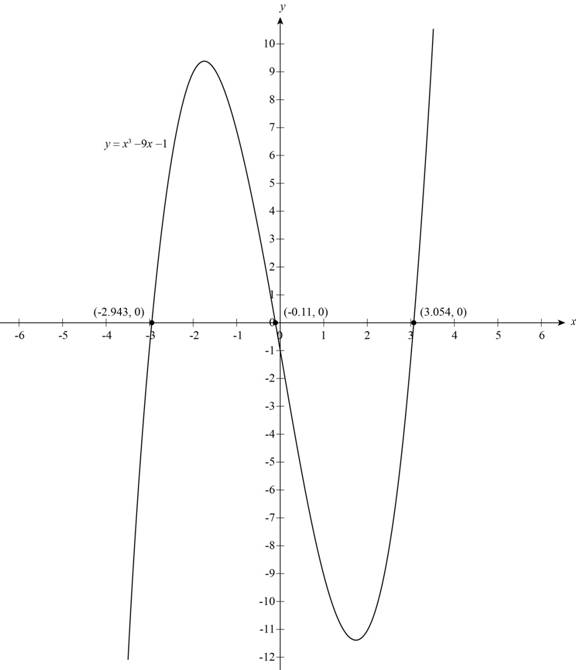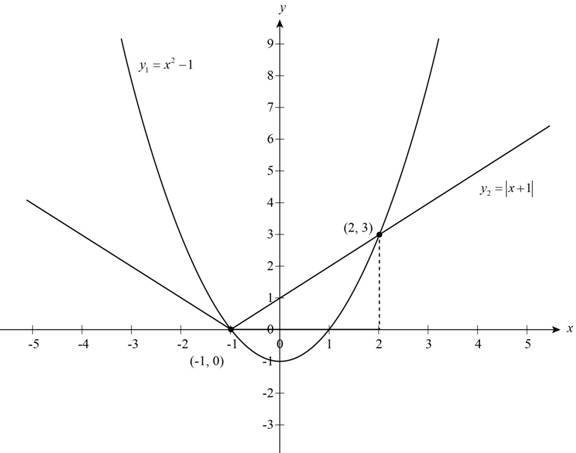# The solution of equation x 3 − 9 x − 1 = 0 through graph.### Precalculus: Mathematics for Calcu...

6th Edition
Stewart + 5 others
Publisher: Cengage Learning
ISBN: 9780840068071### Precalculus: Mathematics for Calcu...

6th Edition
Stewart + 5 others
Publisher: Cengage Learning
ISBN: 9780840068071

#### Solutions

Chapter 1, Problem 14T

(a)

To determine

## To solve: The solution of equation x3−9x−1=0 through graph.

Expert Solution

Solution:

The solutions of equation x39x1=0 are x=2.94 , 0.11 and x=3.05 .

### Explanation of Solution

The given equation is,

x39x1=0

In the above equation let the left-hand side equation is,

y=x39x1

The graphs of equation y is shown below,Figure (1)

The given equation is equivalent to y=0 and the solution is x-coordinate of intersection point of two graphs.

From Figure (1) the graph of y is equal to zero when graph intersect x-axis at x=2.943 , x=0.111 , x=3.054 approximately and these values of x-coordinates give the solution of given equation.

Thus, the solutions of equation x39x1=0 are x=2.94 , 0.11 and x=3.05 .

(b)

To determine

### To solve: The solution of inequality x2−1≤|x+1| through graph.

Expert Solution

The solution of inequality x21|x+1| is the interval [1,2] .

### Explanation of Solution

The given inequality is,

x21|x+1|

In the above equation let left-hand equation is,

y1=x21

The right-hand side equation is,

y2=|x+1|

The graph of equation y1 and y2 on the same set of axis is shown below.Figure (2)

The given equation is equivalent to y1y2 and the solution of inequality is all x-values for which the graph of y1 is less than or equal to y2 .

From Figure (2) the graph of y1 and y2 intersects each other at points (1,0) and (2,3) in which the values of x-coordinates are 1 , 2 and the graph of y1 is on or below the graph y2 when values of x lie in between x=1 to x=2 and these values of x-coordinates give the solution of given inequality.

Thus, the solution of inequality x21|x+1| is the interval [1,2] .

### Have a homework question?

Subscribe to bartleby learn! Ask subject matter experts 30 homework questions each month. Plus, you’ll have access to millions of step-by-step textbook answers!